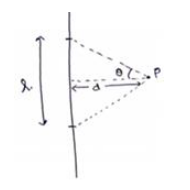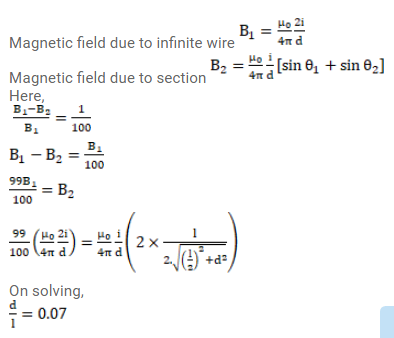# A long, straight wire carries a current i.

Question:

A long, straight wire carries a current i. Let $B_{1}$ be the magnetic field at a point $P$ at a distance d from the wire. Consider a section of length I of this wire such that the point $\mathrm{P}$ lies on a perpendicular bisector of the section. Let $B_{2}$ be the magnetic field at this point due to this section only. Find the value of $d / I$ so that $B_{2}$ differs from $B_{1}$ by $1 \%$.

Solution: